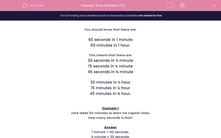# Time Problems (2)

In this worksheet, students must know how many seconds, minutes and hours there are in various time periods, including halves and quarters.This content is premium and exclusive to EdPlace subscribers.Key stage:  KS 2

Curriculum topic:   Maths and Numerical Reasoning

Curriculum subtopic:   Time and Timetables

Difficulty level:#### Worksheet Overview

You should know that there are:

60 seconds in 1 minute

60 minutes in 1 hour.

This means that there are:

30 seconds in ½ minute

15 seconds in ¼ minute

45 seconds in ¾ minute

30 minutes in ½ hour

15 minutes in ¼ hour

45 minutes in ¾ hour.

Example 1

Jack takes 5¼ minutes to learn his capital cities.

How many seconds is this?

1 minute = 60 seconds.

½ minute = 30 seconds.

¼ minute = 15 seconds.

5¼ minutes = 5 × 60 + 15 = 300 + 15 = 315 seconds.

Example 2

Ahmed starts work at 9 a.m. and finishes at 4:30 p.m.

For how many minutes is he at work?

From 9 a.m. to 4 p.m. is 7 hours so Ahmed is at work for 7½ hours.

1 hour = 60 minutes.

½ hour = 30 minutes.

7½ hours = 7 × 60 + 30 = 420 + 30 = 450 minutes.

### What is EdPlace?

We're your National Curriculum aligned online education content provider helping each child succeed in English, maths and science from year 1 to GCSE. With an EdPlace account you’ll be able to track and measure progress, helping each child achieve their best. We build confidence and attainment by personalising each child’s learning at a level that suits them.

Get started